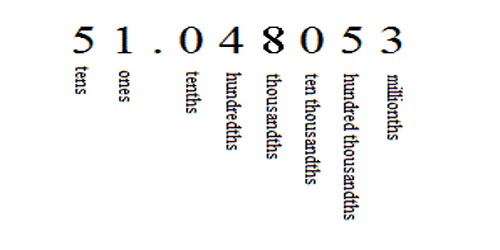Rounding Decimals

Rounding means making a number simpler but keeping its value close to what it was. The result is less accurate, but easier to use. We can round decimals to a certain accuracy or number of decimal places. This is used to make calculation easier to do and results easier to understand, when exact values are not too important.

Example: 73 rounded to the nearest ten is 70, because 73 is closer to 70 than to 80. But 76 goes up to 80.

27.17469 rounded to the nearest whole number is 27

36.74691 rounded to the nearest whole number is 37

12.34690 rounded to the nearest tenth is 12.3

89.46917 rounded to the nearest tenth is 89.5

50.02139 rounded to the nearest hundredth is 50.02

72.63539 rounded to the nearest hundredth is 72.64

46.83531 rounded to the nearest thousandth is 46.835

9.63967 rounded to the nearest thousandth is 9.640Rules for rounding decimals.

1. Retain the correct number of decimal places (e.g. 3 for thousandths, 0 for whole numbers)
2. If the next decimal place value is 5 or more, increase the value in the last retained decimal place by 1.

Rounding Decimals to the Nearest Tenth

Rounding decimals is very similar to rounding other numbers. If the hundredths and thousandths places of a decimal is forty-nine or less, they are dropped and the tenths place does not change.

For example, rounding 0.843 to the nearest tenth would give 0.8.

If the hundredths and thousandths places are fifty or more, the tenths place is increased by one. The decimal 0.866 rounded to the nearest tenth is 0.9.

Rounding Decimals to the Nearest Hundredth

Rounding decimals is very similar to rounding other numbers. If the thousandths place of a decimal is four or less, it is dropped and the hundredths place does not change. For example, rounding 0.843 to the nearest hundredth would give 0.84.

If the thousandths place is five through nine, the hundredths place is increased by one. The decimal 0.846 rounded to the nearest hundredth is 0.85.

Information Source: